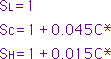###### XYZ Chromaticity Coordinates

The xyz chromaticity coordinates are calculated from the XYZ tristimulus values according to the following formulas:

If the above formulas are used with the X10 Y10 Z10 tristimulus values, the chromaticity coordinates would be x10 y10 z10.

###### xy or x10 y10 Chromaticity Diagram

Two-dimension diagram on which the xy or x10 y10 chromaticity coordinates can be plotted.

###### L*a*b* Color Space

The L*a*b* color space (also referred to as the CIELAB space) is one of the uniform color spaces defined by the CIE in 1976. The values of L*, a*, and b* are calculated according to the formulas below:

###### L*C*h Color Space

The L*C*h color space uses the same diagram as the L*a*b* color space, but uses cylindrical coordinates. Lightness L* is the same as L* in the L*a*b* color space; Metric Chroma C* and Metric Hue-Angle h are defined by the following formulas:

The Metric Hue-Difference is positive if the Metric Hue-Angle h of the specimen is greater than that of the target and negative if the Metric Hue-Angle of the specimen is less than that of the target.

###### Munsell Color System

The Munsell color system consists of a series of color charts which are intended to be used for visual comparison with the specimen. Colors are defined in terms of the Munsell Hue (H; indicates hue), Munsell Value (V; indicates lightness), and Munsell Chroma (C;.indicates saturation) and written as H V/C.

For example, for the color with H=5.0R, V=4.0, and C=14.0, the Munsell notation would be: 5.0R 4.0/14.0

###### Uniform Color Space

A color space in which equal distances on the coordinate diagram correspond to equal perceived color differences.

###### L*u*v Color Space

The L*u*v* color space (also referred to as the CIELUV space) is one of the uniform color spaces defined by the CIE in 1976. The values of L*, u*, and v* are calculated according to the formulas below:

###### CIE 1976 UCS Diagram

CIE 1976 UCS Diagram (for 2° Standard Observer)

The CIE 1976 UCS Diagram was defined by the CIE in 1976. It is intended to provide a perceptually more uniform color spacing for colors at approximately the same luminance. The values of u’ and v’ can be calculated from the tristimulus values XYZ (or X10Y10Z10) or from the chromaticity coordinates xy according to the following formulas:

###### ΔE*94 Color difference formula (CIE 1994)

This color difference formula modifies the lightness, saturation and hue (L*C*h*) of the L*a*b* color space by incorporating factors that correct for variation in perceived color difference magnitude in different areas of CIE 1976 (L*a*b*) Color Space. This was proposed in 1994 by the technical committee of CIE.

Where